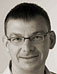# Feedback Controllers

## Exploring the PID controller

In the previous parts of this series (Part 1, Part 2, Part 3, and Part 4), we introduced feedback as a design principle or paradigm, that can help to keep systems “on track”, even in the presence of uncertainty and change. In this post, we will begin to explore more closely what this all means in practice.Consider the feedback loop shown in the Figure. The controlled system is a cache, and we have a controller that adjusts the size of the cache in order to maintain a desired cache hit rate. (Making the cache larger will result in a greater number of hits and hence will drive the hit rate up.) We also have a desired value for the hit rate as reference value or “setpoint” (supplied on the left). The tracking error is calculated as the difference between setpoint and actual hit rate and is provided as input to the controller.

`   tracking error = setpoint - actual`

(The observed hit rate is multiplied by -1 on the return path and then added to the reference value at the circle. Taking both operations together leads to the tracking error, calculated as the difference between the setpoint and the observed value.)

The controller receives the tracking error as input and has to produce a new cache size as output. How, precisely, should it do that? Keep in mind that a negative tracking error (indicating that the actual hit rate is higher than the desired hit rate) should lead to a decrease in the cache size.

The last observation gives us a hint: the momentaneous value of the tracking error can serve as the desired correction to the current cache size! In other words, the control “algorithm” can be summarized in the following one-liner:

`  new size = old size + k * tracking error`

If the tracking error is negative (meaning that the actual hit rate is larger than it should be), the cache size will be reduced; if the tracking error is positive (because the actual hit rate
is too small), then the cache size will be increased.

The factor k here is a numerical factor, which is required to adjust the different “scales”: since the hit rate is a number between 0.0 and 1.0, the tracking error itself is also a floating-point number with absolute magnitude between 0.0 and 1.0. But the cache size, that is the maximum number of items in the cache, is an integer, which might be quite large, depending on the nature of the request traffic. (How many distinct items are typically being requested?) The “gain factor” k takes this difference of scales into account, but also allows us to adjust (or “tune”) the loop to its specific environment. It’s through the controller gain k that we can adapt the same controller to work in a situation where 100 documents make up the 90 percent of requested items, and to a situation where 100,000 documents constitute the same fraction of traffic.

The question of choosing the controller gain aside, it is worth dwelling on the structure of the control algorithm for a moment. By construction, it works in both directions: no matter whether the current hit rate is too high or too low, the updating formula works in either case. Moreover, because the correction is proportional to the tracking error, the formula has the nice property that larger errors automatically lead to larger corrections. And finally, if the tracking error vanishes (because the actual hit rate is exactly equal to the desired hit rate), the correction vanishes as well, and the cache size no longer changes. Just as it should.

Given all this, it will come as no surprise that the simple formula above is used in the majority of feedback controllers, but it is often augmented by additional contributions. The most popular controller is the aptly-named “three-term” or PID (for “proportional, integral, derivative”) controller. The output of a PID controller depends on:

• the current value of the error (the presence)
• the total, cumulative sum of all errors to date (the past)
• the most recent change in the error (the predicted future)

Mathematically speaking, the cumulative “sum” is replaced by an integral, and the most recent “change” is given by the derivative, so that the full formula for the output of a PID controller is given by:Here, the three controller gains kp, ki, and kd are three numerical factors, and e(t) is the current value of the error. Often, the derivative term is not used (in other words, one often sets kd = 0). If we set kp = 0 as well, this formula reduces to the one-liner shown earlier.

In a computer implementation, time does not progress continuously, but in discrete steps (of size DT). An implementation of the PID controller would then look like this:

```sum += error
output = kp * error + DT * ki * sum + kd * (error - prev)/DT
prev = error```

This is it! This is all there is to the famous “three-term” or PID controller! This is the controller that is (by some studies) being used in over 90 percent of all industrial installations.

We see here an important aspect of many feedback systems: feedback control trades a more complicated architecture for a simpler controller. Feedback systems work, not because the controller itself is clever, but because of the way that information is looped around and “fed back” into the controller.

The other important question that should be on everybody’s mind now concerns the three controller gains, kp, ki, and kd. How do we choose them? That will be the topic of our next post. Stay tuned!

tags: ,